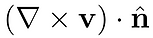top of page

## Definition of divergence

Rather than introducing the divergence of a vector field w as an inner product between w and the "vector" del, here we define the divergence in a way that physically makes more sense.The divergence of w at point P is defined asAnd it turns out that this is equal toThe divergence of a vector is a measure of how much the vector spreads out from the point in question.

## Definition of curl

To define the curl of a vector field v at point P, we consider a circle with area whose center is at P.The n component of the curl of v at point P is defined asIt turns out that this is equal toSpecifically,The curl of a vector is a measure of how much the vector curls around the point in question.

bottom of page